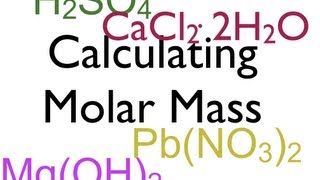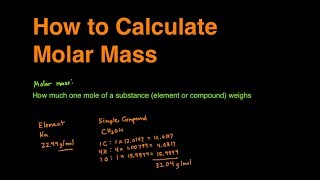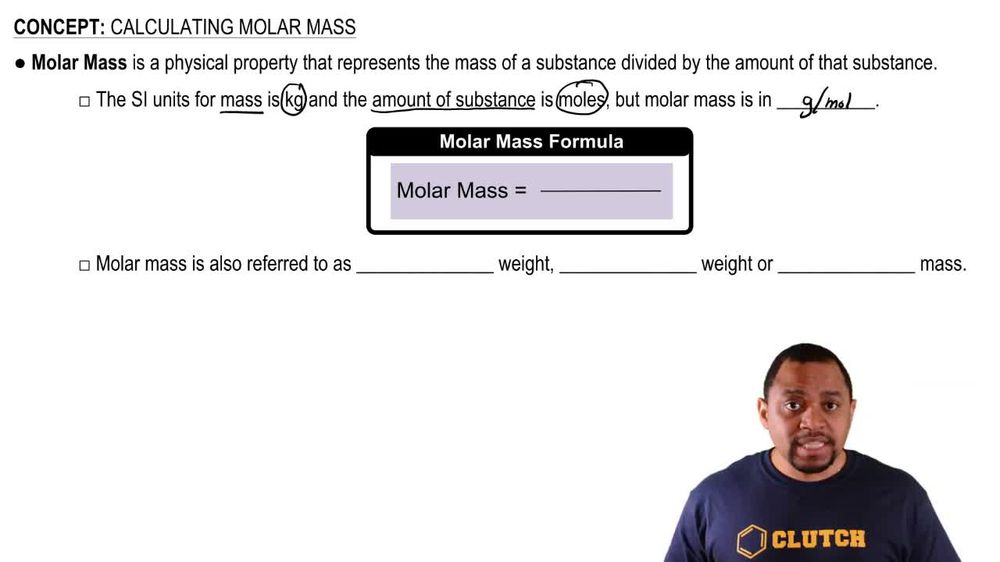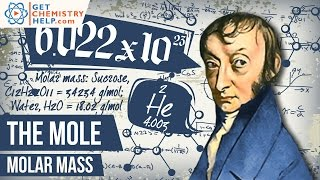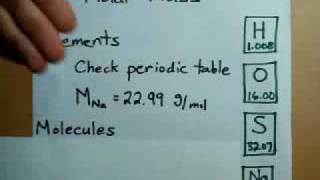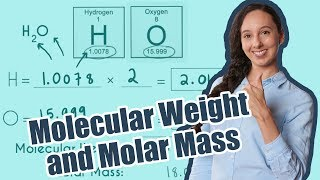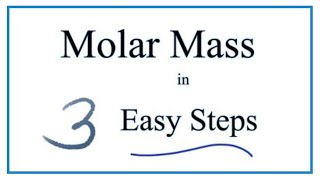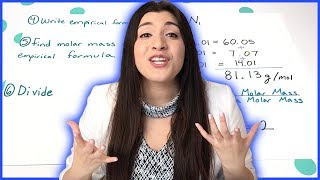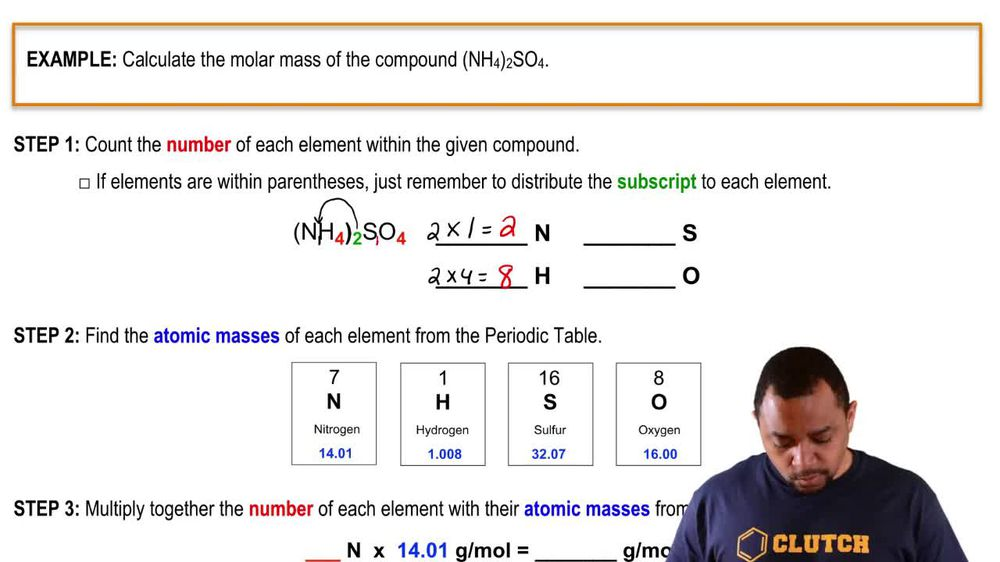Start typing, then use the up and down arrows to select an option from the list.
1. 2. Atoms & Elements2. Calculating Molar Mass
Problem

# What are the molecular (formula) weights of the following substances? (b) C4H8O2 (butyric acid, responsible for the odor of rancid butter)

Relevant Solution1m
Play a video:
Hey everyone in this example, we need to determine the formula mass of Anthony like acid. We want to recall that formula mass is interchangeable with the term atomic mass. So we're going to go ahead and calculate based on each amount of atoms we have in this compound, our formula mass. So for carbon we want to recognize that we have that subscript of seven and so we have seven times Or seven atoms times its atomic mass according to our periodic table equal to 12.01 am use. This gives us a value equal to 84.07 AM. You next? We have our hydrogen atom which we see we have seven atoms of as well which we can multiply by hydrogen atomic masses on the periodic table of 1.8 AM use And this is going to give us 7.056 am use. Then we have our nitrogen atom which we only have one of because we don't have any subscript next to it. So we would say one times its atomic mass according to the periodic table of 14.1 A. M. U. S. Which gives us the same thing 14.01 a. m. use. And lastly we have our oxygen in which we have two atoms of so we take two times its atomic mass according to the periodic table of 16 AM Use. And that gives us a total of 32 a. m. use. And so we're going to take the total of all of the contributions of atomic mass units from each of these atoms. And this is going to give us a total of 137.14 am use as our formula mass of our Anthony Illich assets. So C seven H seven N 02. So this is our final answer for this example, if you have any questions, please leave them down below and I will see everyone in the next practice video.